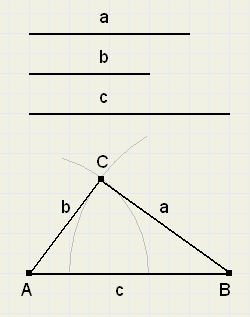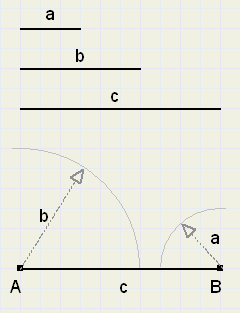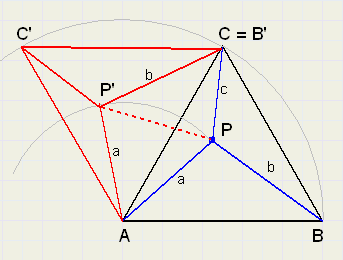Triangles and SidesProblem
Given are 3 lines with lengths a, b and c.
Which conditions enable the construction of a triangle having a,b and c as sides?

Refer to figure 1. below.
The triangle has c as base. Angle C is the intersection of the circles with radii a and b,
and centres A and B.fig.1

now look at figure 2.fig.2

In fig.1, the construction of a triangle was possible. In fig.2 it is not.
The reason is clear : in fig. 2 , a en b together are smaller than c.

De condition therefore can be written as the inequality :
c < a + b
Of course, also must be valid::
a < b + c .............and
b < a + c
The following is funny:

If we call s half the perimeter of the triangle, then:
a + b + c = 2s

starting with the inequality

a < b + c...............we may write (left and right : add a)
2a < a + b + c
2a < 2s.................so
a < s
This should be true for b and c as well, so we state:
every side of a triangle must be smaller then half of the perimeter
Another nice problem
Given is a equilateral triangle containing an arbitrary point P.
From P, we add lines PA = a, PB = b and PC = c.

Prove, that a, b and c always may be sides of a triangle.
See figure 3 below:fig.3

Solution 1.
ABC is equilateral, assume it's sides have length z.
We observe:
a < z ...................and also
z < b + c
...........................combining:
a < b + c
and similar:
b < a + c
c < a + b
Which concludes the proof.

Solution 2.
We rotate the triangle by 60 degrees, A being the centre of the rotation.
So B arrives in C, C becomes C' en P becomes P'. see figure 4.fig.4

Triangle APP' is equilateral because LP'AP is 60 degrees and P'A = PA = a,
so LPP'A = LP'PA = 60 degrees.
So PP'= a and we notice that triangle CPP' has sides a,b and c.
Quite surprising.